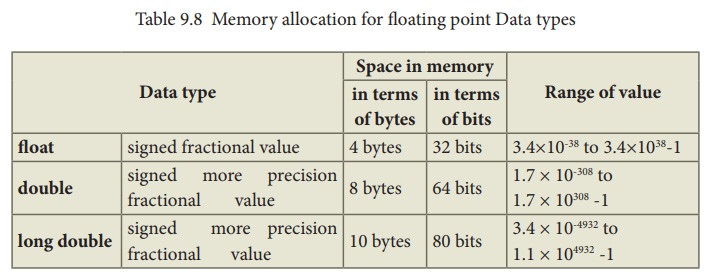Home | | Computer Science 11th std | Data type modifiers

# Data type modifiers

Modifiers can be used to modify (expand or reduce) the memory allocation of any fundamental data type. They are also called as Qualifiers.

Data type modifiers:

Modifiers are used to modify the storing capacity of a fundamental data type except void type. Usually, every fundamental data type has a fixed range of values to store data items in memory. For example, int data type can store only two bytes of data. In reality, some integer data may have more length and may need more space in memory. In this situation, we should modify the memory space to accommodate large integer values. Modifiers can be used to modify (expand or reduce) the memory allocation of any fundamental data type. They are also called as Qualifiers.There are four modifiers used in C++. They are:

(1) signed

(2) unsigned

(3) long

(4) short

These four modifiers can be used with any fundamental data type. The following Table 9.6 shows the memory allocation for each data type with and without modifiers.

## Integer type

Table 9.6 Memory allocation for Data typesThe above table clearly shows that an integer type accepts only 2 bytes of data whereas a long int accepts data that is double this size i.e., 4 bytes of data. So, we can store more digits in a long int. (long is modifier and int is a fundamental data type)

## char type

Table 9.7 Memory allocation for char Data types## Floating point type

Table 9.8 Memory allocation for floating point Data typesMemory allocation is subjected to vary based on the type of compiler that is being used. Here, the given values are as per the Turbo C++ compiler. Dev C++ provides some more space to int and long double types. Following Tables 9.9 shows the difference between Turbo C++ and Dev C++ allocation of memory.

Table 9.9 Memory allocation by Turbo C++ and Dev C++Since, Dev C++ provides 4 bytes to int and long, any one of these types can be used to handle bigger integer values while writing programs in Dev C++.

Note: sizeof( ) is an operator which gives the size of a data type.

## Illustration 9.5: C++ Program to find the size of data types

#include <iostream>

using namespace std;

int main()

{

short a;

nsigned short b;

signed short c;

int d;

unsigned int e;

signed int f;

long g;

unsigned long h;

signed long i;

char j;

unsigned char k;

signed char l;

float m;

double n;

long double p;

cout << "\n Size of short = " << sizeof(a);

cout << "\n Size of unsigned short = " << sizeof(b);

cout << "\n Size of signed short = " << sizeof (c);

cout << "\n Size of int = " << sizeof(d);

cout << "\n Size of unsigned int = " << sizeof(e);

cout << "\n Size of signed int = " << sizeof(f);

cout << "\n Size of long = " << sizeof(g);

cout << "\n Size of unsigned long = " << sizeof(h);

cout << "\n Size of signed long = " << sizeof(i);

cout << "\n Size of char = " << sizeof(j);

cout << "\n Size of unsigned char = " << sizeof(k);

cout << "\n Size of signed char = " << sizeof(l);

cout << "\n Size of float = " << sizeof(m);

cout << "\n Size of double = " << sizeof(n);

cout << "\n Size of long double = " << sizeof(p);

}

### Output : (compiled and executed in Dev C++)

Size of short = 2

Size of unsigned short = 2

Size of signed short = 2

Size of int = 4

Size of unsigned int = 4

Size of signed int = 4

Size of long = 4

Size of unsigned long = 4

Size of signed long = 4

Size of char = 1

Size of unsigned char = 1

Size of signed char = 1

Size of float = 4

Size of double = 8

Size of long double = 12

## Number Suffixes in C++

There are different suffixes for integer and floating point numbers. Suffix can be used to assign the same value as a different type. For example, if you want to store 45 in an int, long, unsigned int and unsigned long int, you can use suffix letter L or U (either case) with 45 i.e. 45L or 45U. This type of declaration instructs the compiler to store the given values as long and unsigned. ‘F’ can be used for floating point values, example: 3.14F

Tags : Introduction to C++ , 11th Computer Science : Chapter 9 : Introduction to C++
Study Material, Lecturing Notes, Assignment, Reference, Wiki description explanation, brief detail
11th Computer Science : Chapter 9 : Introduction to C++ : Data type modifiers | Introduction to C++